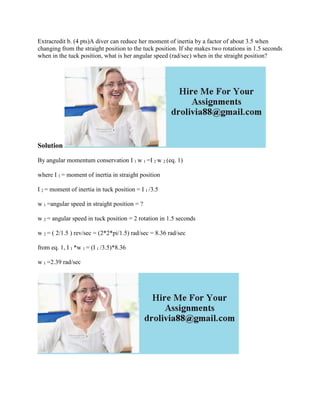Seu SlideShare está sendo baixado. ×

# Extracredit b- (4 pts)A diver can reduce her moment of inertia by a fa.docx

Anúncio
Anúncio
Anúncio
Anúncio
Anúncio
Anúncio
Anúncio
Anúncio
Anúncio
Anúncio
AnúncioCarregando em…3
×

1 de 1 Anúncio

# Extracredit b- (4 pts)A diver can reduce her moment of inertia by a fa.docx

Extracredit b. (4 pts)A diver can reduce her moment of inertia by a factor of about 3.5 when changing from the straight position to the tuck position. If she makes two rotations in 1.5 seconds when in the tuck position, what is her angular speed (rad/sec) when in the straight position?
Solution
By angular momentum conservation I 1 w 1 =I 2 w 2 (eq. 1)
where I 1 = moment of inertia in straight position
I 2 = moment of inertia in tuck position = I 1 /3.5
w 1 =angular speed in straight position = ?
w 2 = angular speed in tuck position = 2 rotation in 1.5 seconds
w 2 = ( 2/1.5 ) rev/sec = (2*2*pi/1.5) rad/sec = 8.36 rad/sec
from eq. 1, I 1 *w 1 = (I 1 /3.5)*8.36
.

Extracredit b. (4 pts)A diver can reduce her moment of inertia by a factor of about 3.5 when changing from the straight position to the tuck position. If she makes two rotations in 1.5 seconds when in the tuck position, what is her angular speed (rad/sec) when in the straight position?
Solution
By angular momentum conservation I 1 w 1 =I 2 w 2 (eq. 1)
where I 1 = moment of inertia in straight position
I 2 = moment of inertia in tuck position = I 1 /3.5
w 1 =angular speed in straight position = ?
w 2 = angular speed in tuck position = 2 rotation in 1.5 seconds
w 2 = ( 2/1.5 ) rev/sec = (2*2*pi/1.5) rad/sec = 8.36 rad/sec
from eq. 1, I 1 *w 1 = (I 1 /3.5)*8.36